Search

Systems of Non-Linear Equations:
Graphical Considerations
(page 2 of 6)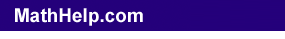The previous page refreshed us on the relationship between solutions of equations and the points on the associated plots of those equations' lines. This topic was probably last mentioned in class back when you first learned about graphing linear equations, and may have been forgotten since. But the issue is important for solving systems of equations. Why? Because the solution to a system of equations is going to be a solution for all of the equations in the system. Then that solution must be a plot point for all of the associated lines' graphs. But where will different lines have the same plotted point? Where those lines intersect.

Suppose you have the following system of equations:

• Solve the system by graphing:
• y = x2
y
= 8  x2

 I can graph each of these equations separately:   y = x2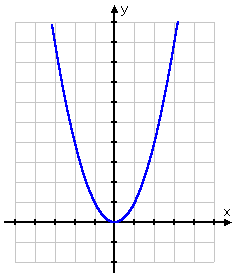y = 8  x2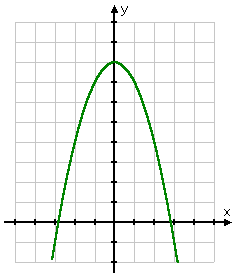..and each point on each graph is a solution to that graph's equation.

 Now look at the graph of the system: y = x2 y = 8  x2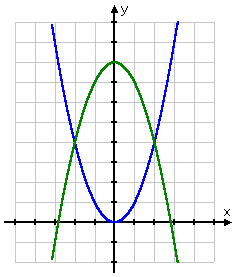A solution to the system is any point that is a solution for both equations. In other words, a solution point for this system is any point that is on both graphs. In other words:

"SOLUTIONS" FOR SYSTEMS
ARE
INTERSECTIONS OF THE LINES

 Then, graphically, the solutions for this system are the red-highlighted points at right: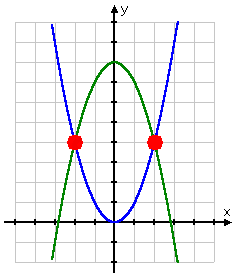That is, the solutions to this system are the points (2, 4) and (2, 4).

So when you're trying to solve a system of equations, you're trying to find the coordinates of the intersection points.  Copyright © 2002-2011 Elizabeth Stapel All Rights Reserved

Content Continues Below

The system in the above example had two solutions, because the graph shows two intersection points. Different systems can have different numbers of solutions. For instance:

 A system can have one solution: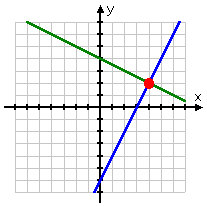...lots of solutions: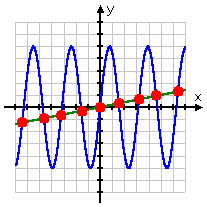...or no solutions at all: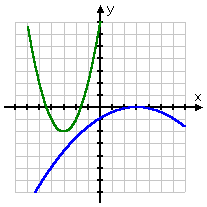(In this last situation, where there was no solution, the system of equations is said to be "inconsistent".)

 When you look at a graph, you can only guess at an approximation to the solution. Unless the solutions points are nice neat numbers (and unless you happen to know this in advance), you can't get the solution from the picture. For instance, you can't tell what the solution to the system graphed at right might be: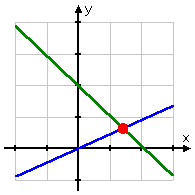...because you're having to guess from a picture. As it happens, the solution is (x, y) = (13/7,  9/14), but you would have no possible way of knowing that from this picture.

Advisory: Your text will almost certainly have you do some "solve by graphing" exercises. You may safely assume for these exercises that answers are nice and neat, because the solutions must be if you are to be able to have a chance at guessing the solutions from a picture.

This "solving by graphing" can be useful, in that it helps you get an idea in picture form of what is going on when solving systems. But it can be misleading, too, in that it implies that all solutions will be "neat" ones, when most solutions are actually rather messy.

<< Previous  Top  |  1 | 2 | 3 | 4 | 5 | 6  |  Return to Index  Next >>

 Cite this article as: Stapel, Elizabeth. "Systems of Non-Linear Equations: Graphical Considerations." Purplemath.     Available from https://www.purplemath.com/modules/syseqgen2.htm.     Accessed [Date] [Month] 2016

 Copyright © 2021  Elizabeth Stapel   |   About   |   Terms of Use   |   Linking   |   Site Licensing Contact Us